# Estimate Neural State-Space Model

Estimate neural state-space model in the Live Editor

Since R2023b

## Description

The Estimate Neural State-Space Model task is independent of the more general System Identification app. Use the System Identification app when you want to compute and compare estimates for multiple model structures.

To get started, load experiment data that contains input and output data into your MATLAB workspace and then import that data into the task. Then specify a model structure to estimate. The task gives you controls and plots that help you experiment with different model parameters and compare how well the output of each model fits the measurements.

### Related Functions

The code that Estimate Neural State-Space Model generates uses the following functions and objects.

The task estimates an `idNeuralStateSpace` state-space model.

To add the Estimate Neural State-Space Model task to a live script in the MATLAB Editor:

• On the Live Editor tab, select Task > Estimate Neural State-Space Model.

• In a code block in your script, type a relevant keyword, such as `neuralstatespace` or `nlssest`. Select `Estimate State-Space Model` from the suggested command completions.

## Examples

expand all

Use the Estimate Neural State-Space Model Live Editor Task to estimate a neural state-space model and compare the model output with the measurement data.

Open this example to see a preconfigured script containing the task.

Generate Data

For this example, generate data by simulating a first-order linear system. First, fix the random generator seed to guarantee reproducibility.

`rng(0)`

Create a first-order discrete dynamical system in `tf` form with one input and one output, convert it to discrete time using a sample time of 0.1 sec, and use `ss` to obtain a state-space realization.

```Ts = 0.1; sys = ss(c2d(tf(1,[1 1]),Ts));```

The identification of a neural state-space system requires you to have measurement of the system states. Therefore, transform the state-space coordinates so that the output is equal to the state. Alternatively you can augment the output equation to include the state among the measured signals.

```sys.b = sys.b*sys.c; sys.c = 1;```

In general, it is good practice to use multiple experiments, each containing a different trajectory, as doing so is more likely to yield a better coverage of the state-input space. Furthermore, using long trajectories tends to reduce both the accuracy and efficiency of the estimation. However, for this example, use a single trajectory for estimation.

Define a time vector and a random input sequence for estimation (training).

```te = 0:Ts:10; ue = randn(length(te),size(sys.B,2));```

Generate an output response to the random input sequence by simulating the system from a zero initial condition. The first (vertical) dimension in `ye` must be time and the second (horizontal) dimension must be the specific output in the output vector signal.

`ye = lsim(sys,ue,te,zeros(size(sys.B,1),1));`

Define a shorter time vector and a random input sequence for validation.

```tv = 0:Ts:1; uv = randn(length(tv),size(sys.B,2));```

Generate an output response to the random input sequence by simulating the system, from a zero initial condition.

`yv = lsim(sys,uv,tv,zeros(size(sys.B,1),1));`

In the Select data section, set Data Type to `Numeric`, Sample Time to `0.01`, Estimation Data: Input (u) to `ue`, Estimation Data: Output (y) to `ye`, Validation Data: Input (u) to `uv`, and Validation Data: Output (y) to `yv`.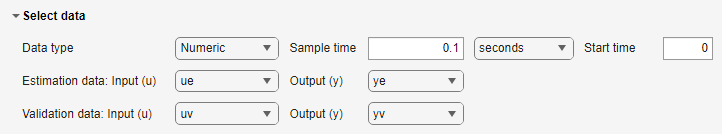Specify Model Structure and State Network

In the Specify model structure section, set the Number of states to 1 and select the discrete-time domain. In the State network section, set the Number of states to 1 and specify Layer size as 16. Leave the other options unchanged.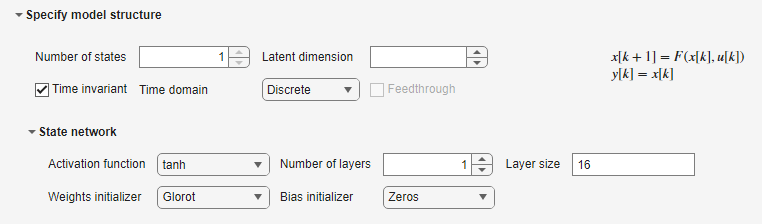Note that since for this example the output is equal to the state, there is no Output network section.

Examine Training and Display Options

In the Specify training options section, the Training algorithm is set to `ADAM`, with a Learn rate of `0.001`. The number of epochs is set to `100`, and so is the mini-batch size. For more information on these options, see `nssTrainingOptions`.

In the Display results section, both the Show fit to estimation data and (since you have specified validation data) the Show fit to validation data are selected.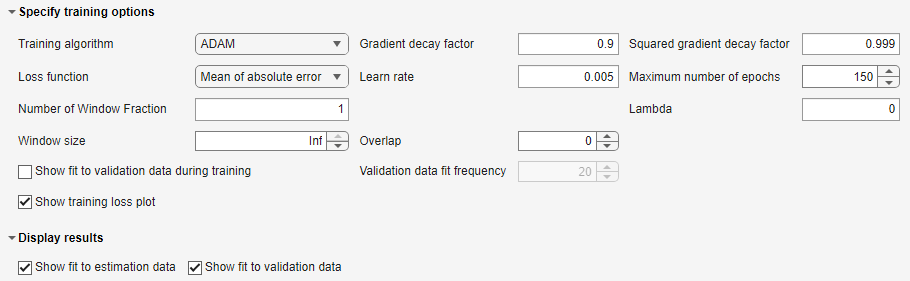Execute the task from the Live Editor tab using Run. During training, a plot displays the training losses of the state and output networks.`Generating estimation report...done.`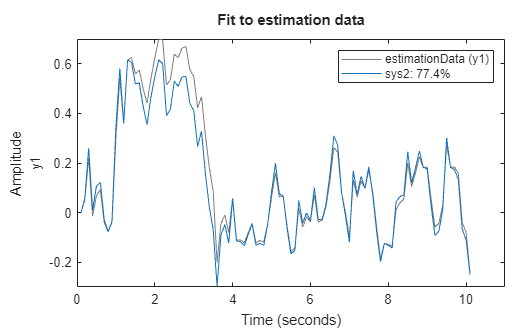After training, two plots displays the model fit on the estimation and validation data.

Generate Code

To display the code that the task generates, click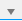(Show code) at the bottom of the parameter section. The code that you see reflects the current parameter configuration of the task.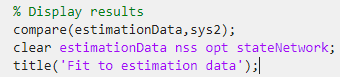## Parameters

expand all

Select data

The task accepts numeric measurement values that are uniformly sampled in time. Input and output signals can contain multiple channels. Data can be packaged as numeric arrays, in an `iddata` object, or in a `timetable` object. For multiexperiment data, numeric and timetable data can be packaged as cell arrays. For cell arrays of timetables, all timetables must contain the same variable names. Data objects handle multiexperiment data internally.

The data type you choose determines whether you must specify additional parameters.

• `Numeric` — Specify Sample Time and Start Time in the time unit that you select. Additionally, you need to specify different workspace variables containing the input and output signals to be used for estimation and (if available) validation.

• `Timetable` — Specify no additional parameters because the timetable already contains the input and output signals and sampling information.

• `iddata object` — Specify no additional parameters because the `iddata` object already contains the input and output signals and sampling information.

Sample time at which estimation and (if available) validation data are collected, specified as a positive scalar, in the unit specified by the following time unit drop down list. You can specify a sample time only when Data Type is `Numeric`.

Time unit for the Sample time and Start time parameters. You can specify one of the following units:

• `nanoseconds`

• `microseconds`

• `milliseconds`

• `seconds`

• `minutes`

• `hours`

• `days`

• `weeks`

• `months`

• `years`

You can specify a sample time only when Data Type is `Numeric`.

Start time for the estimation and (if available) validation data, specified as a nonnegative scalar, in the unit specified by the preceding time unit drop down list. This value is relevant only if you deselect the Time invariant checkbox. You can specify a start time only when Data Type is `Numeric`.

Name of the input data variable used for estimation, selected from the MATLAB workspace choices. Use this parameter, along with Estimation data: Output (y), when Data Type is `Numeric`.

Name of the output data variable used for estimation, selected from the MATLAB workspace choices. Use this parameter, along with Estimation data: Input (u), when Data Type is `Numeric`.

Select the timetable object variable name from the MATLAB workspace choices. If you use a use a cell arrays of timetables, all timetables must contain the same variable names. Use this parameter when Data type is `Timetable`.

Select the `iddata` object variable name from the MATLAB workspace choices. Use this parameter when Data type is `iddata object`.

Name of the input data variable used for validation, selected from the MATLAB workspace choices. Use this parameter, along with Validation data: Output (y), when Data Type is `Numeric`.

Name of the output data variable used for validation, selected from the MATLAB workspace choices. Use this parameter, along with Validation data: Input (y), when Data Type is `Numeric`.

Select the timetable object variable name from the MATLAB workspace choices. The timetables containing the validation data must have the same variable names as the ones in the timetables selected for estimation in Estimation data: Timetable. Use this parameter when Data type is `Timetable`. Specifying validation data is optional but recommended.

Select the `iddata` object variable name from the MATLAB workspace choices. Use this parameter when Data type is `iddata object`. Specifying validation data is optional but recommended.

Specify model structure

Number of states in the model to estimate. It must be less than or equal to the number of outputs in the data. For more information, see `idNeuralStateSpace`.

Deselect this option to estimate a model in which the state equation explicitly depends on time, other than states and inputs. When this option is left selected (default), the state equation depends explicitly only on the current state and input vectors. For more information, see the `isTimeInvariant` property of `idNeuralStateSpace`.

Select a continuous-time or discrete-time model.

Select this option to estimate a model in which the output equation explicitly depends on the input vector. When this option is left unselected (default), the output equation does not depend explicitly on the input vector. For more information, see the `HasFeedthrough` property of `idNeuralStateSpace`.

State network

You can specify one of the following:

• `tanh` — Use the hyperbolic tangent activation function for all hidden layers of the state network (default).

• `sigmoid` —Use the sigmoid activation function for all hidden layers of the state network.

For more information, see `createMLPNetwork`.

Number of hidden layers of the state network, specified as a nonnegative integer. It must be equal to the number of elements of the vector you specify in Layer size in the State network section. If you specify `0`, the state network has no hidden layer, and therefore expresses a linear function.

For more information, see `createMLPNetwork`.

Size of the hidden layers for the state network, specified as a vector of positive integers. Each number specifies the number of neurons (network nodes) for each hidden layer (each layer is fully-connected). For example, `[10 20 8]` specifies a network with three hidden layers, the first (after the network input) having `10` neurons, the second having `20` neurons, and the last (before the network output), having `8` neurons. Note that the output layer is also a fully-connected, and you cannot change its size.

The number of elements in Layer size must be equal to the value specified in Number of layers in the State network section.

For more information, see `createMLPNetwork`.

Weights initializer method for all the hidden layers of the state network. You can specify one of the following:

• `glorot` — Use the Glorot method (default).

• `he` — Use the He method.

• `orthogonal` — Use the orthogonal method.

• `narrow-normal` — Use the narrow-normal method.

• `zeros` — Initialize all weights to zero.

• `ones` — Initialize all weights to one.

For more information, see `createMLPNetwork`.

Bias initializer method for all the hidden layers of the state network. You can specify one of the following:

• `zeros` — Initializes all biases to zero (default).

• `ones` — Initializes all biases to one.

• `narrow-normal` — uses the narrow-normal method.

For more information, see `createMLPNetwork`.

Output network

You can specify one of the following:

• `tanh` — Use the hyperbolic tangent activation function for all hidden layers of the output network (default).

• `sigmoid` —Use the sigmoid activation function for all hidden layers of the output network.

For more information, see `createMLPNetwork`.

Number of hidden layers of the output network, specified as a nonnegative integer. It must be equal to the number of elements of the vector you specify in Layer size in the Output network section. If you specify `0`, the output network has no hidden layer, and therefore expresses a linear function.

For more information, see `createMLPNetwork`.

Size of the hidden layers for the output network, specified as a vector of positive integers. Each number specifies the number of neurons (network nodes) for each hidden layer (each layer is fully-connected). For example, `[10 20 8]` specifies a network with three hidden layers, the first (after the network input) having `10` neurons, the second having `20` neurons, and the last (before the network output), having `8` neurons. Note that the output layer is also a fully-connected, and you cannot change its size.

The number of elements Layer size must be equal to the value specified in Number of layers in the Output network section.

For more information, see `createMLPNetwork`.

Weights initializer method for all the hidden layers of the output network. You can specify one of the following:

• `glorot` — Use the Glorot method (default).

• `he` — Use the He method.

• `orthogonal` — Use the orthogonal method.

• `narrow-normal` — Uses the narrow-normal method.

• `zeros` — Initializes all weights to zero.

• `ones` — Initializes all weights to one.

For more information, see `createMLPNetwork`.

Bias initializer method for all the hidden layers of the output network. You can specify one of the following:

• `zeros` — Initialize all biases to zero (default).

• `ones` — Initialize all biases to one.

• `narrow-normal` — Use the narrow-normal method.

For more information, see `createMLPNetwork`.

ODE Solver options

Initial step size used to simulate the model (when continuous-time). It is specified as either `Auto` or a positive scalar. If you specify `Auto`, then the solver bases the initial step size on the slope of the solution at the initial time point.

For more information, see `odeset`, `nssTrainingADAM` and `nssTrainingSGDM`.

Maximum step size used to simulate the model (when continuous-time). It is an upper bound on the size of any step taken by the solver, and it is specified as either `Auto` or a positive scalar. If you specify `Auto`, then the value used is one-tenth of the difference between final and initial time.

For more information, see `odeset`, `nssTrainingADAM` and `nssTrainingSGDM`.

Absolute tolerance used to simulate continuous time models, specified as a positive scalar. It is the largest allowable absolute error. That is, when the solution approaches 0, `AbsoluteTolerance` is the threshold below which you do not worry about the accuracy of the solution since it is effectively 0.

For more information, see `odeset`, `nssTrainingADAM` and `nssTrainingSGDM`.

Relative tolerance used to simulate the continuous time models, specified as a positive scalar. This tolerance measures the error relative to the magnitude of each solution component. That is, it controls the number of significant digits in a solution (except when is smaller than the absolute tolerance).

For more information, see `odeset`, `nssTrainingADAM` and `nssTrainingSGDM`.

Specify training options

You can specify one of the following:

• `ADAM` — use the Adam (name derived from adaptive moment estimation) algorithm.

• `SGDM` —use the SGDM (stochastic gradient descent with momentum) algorithm.

For more information, see `nssTrainingOptions`.

Decay rate of gradient moving average for the Adam solver, specified as a nonnegative scalar less than `1`. The default value works well for most tasks. You can specify a Gradient decay factor only when Training algorithm is `ADAM`.

For more information, see `nssTrainingADAM` and `TrainingOptionsADAM` (Deep Learning Toolbox).

Decay rate of squared gradient moving average for the Adam solver, specified as a nonnegative scalar less than `1`.

Typical values of the decay rate are `0.9`, `0.99`, and `0.999`, corresponding to averaging lengths of `10`, `100`, and `1000` parameter updates, respectively. You can specify a Gradient decay factor only when Training algorithm is `ADAM`.

For more information, see `nssTrainingADAM` and `TrainingOptionsADAM` (Deep Learning Toolbox).

Contribution of the parameter update step of the previous iteration to the current iteration of the SGDM algorithm, specified as a scalar from `0` to `1`.

A value of `0` means no contribution from the previous step, whereas a value of `1` means maximal contribution from the previous step. The default value works well for most tasks. You can specify a Gradient decay factor only when Training algorithm is `SGDM`.

For more information, see `nssTrainingSGDM` and `TrainingOptionsSGDM` (Deep Learning Toolbox).

Example: `0.9`

You can specify one of the following:

• `Mean of absolute error` — Use the mean value of the absolute error.

• `Mean of square error` — Use the mean value of the squared error.

For more information, see `nssTrainingADAM` and `nssTrainingSGDM`.

Learning rate used for training, specified as a positive scalar. If the learning rate is too small, then training can take a long time. If the learning rate is too large, then training might reach a suboptimal result or diverge.

For more information, see `nssTrainingADAM` and `nssTrainingSGDM`.

Maximum number of epochs to use for training, specified as a positive integer. An epoch is the full pass of the training algorithm over the entire training set.

For more information, see `nssTrainingADAM` and `nssTrainingSGDM`.

Size of the mini-batch to use for each training iteration, specified as a positive integer. A mini-batch is a subset of the training set that is used to evaluate the gradient of the loss function and update the weights.

If the mini-batch size does not evenly divide the number of training samples, then the estimation process discards the training data that does not fit into the final complete mini-batch of each epoch.

For more information, see `nssTrainingADAM` and `nssTrainingSGDM`.

You can select one of the following options:

• `zoh` — Zero-order hold interpolation method

• `foh` — First-order hold interpolation method

• `cubic` — Cubic interpolation method

• `makima` — Modified Akima interpolation method

• `pchip` — Shape-preserving piecewise cubic interpolation method

• `spline` — Spline interpolation method (default)

This is the interpolation method used to interpolate the input when integrating continuous-time neural state-space models. For more information, see interpolation methods in `interp1`.

Example: `"foh"`

Enable displaying a validation plot periodically during training. The validation plot shows a comparison between the predicted output response to measured validation inputs and the measured validation outputs. The plot also displays the model fit percentage.

This is the number of epochs after which the validation plot is updated with a new comparison (new predicted output against measured outputs). For example, if Validation data fit frequency is 10, the validation plot is updated every 10 epochs. For more information, see `nlssest`.

Enable displaying a training plot during training (estimation). The training plot shows how the state and output network loss values evolve after each training epoch.

Display results

After estimation (training), plot a comparison between the predicted output response to measured estimation inputs and the measured estimation outputs. Selecting this parameter also displays the model fit percentage.

After estimation (training), plot a comparison between the predicted output response to measured validation inputs and the measured validation outputs. Selecting this parameter also displays the model fit percentage. This parameter is available only if you select validation data in the Select Data section.

## Version History

Introduced in R2023b